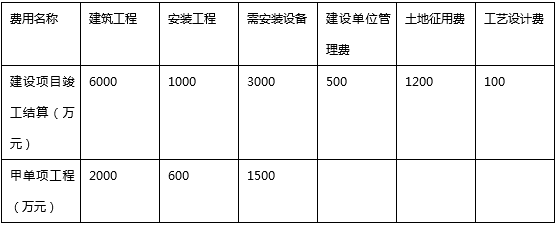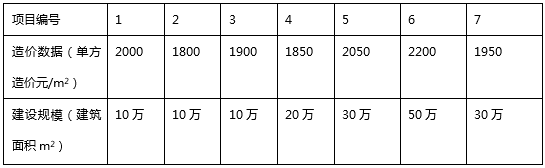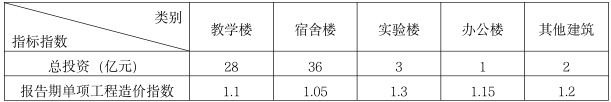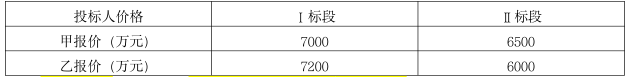83:002022年《建设工程计价》真题

1
(单项选择题)

• A.

直接费

• B.

间接费

• C.

暂定金额

• D.

分包费

• A
• B
• C
• D

2
(单项选择题)

• A.

财务专用工具购置费

• B.

预付款担保

• C.

审计费

• D.

财产保险费

• A
• B
• C
• D

3
(单项选择题)

• A.

预算定额

• B.

概算定额

• C.

概算指标

• D.

投资估算指标

• A
• B
• C
• D

4
(单项选择题)

• A.

安全文明施工费

• B.

暂列金额

• C.

计日工

• D.

规费

• A
• B
• C
• D

5
(单项选择题)

• A.

可行性研究费

• B.

节能评估费

• C.

设计评审费

• D.

技术经济标准使用费

• A
• B
• C
• D

6
(单项选择题)

• A.

1%

• B.

1.5%

• C.

3%

• D.

5%

• A
• B
• C
• D

7
(单项选择题)

• A.

预算单价法

• B.

扩大单价法

• C.

设备价值百分比法

• D.

综合吨位指标法

• A
• B
• C
• D

8
(单项选择题)

• A.

如附着物产权属个人，则该项补助费付给个人。

• B.

应向土地受让者征收契税

• C.

转让土地如有增值，要向受让者征收土地增值税。

• D.

土地使用者按规定的标准一次性缴纳土地使用费。

• A
• B
• C
• D

9
(单项选择题)

• A.

全面审查

• B.

重点审查

• C.

抽样审查

• D.

对比审查与抽样审查相结合

• A
• B
• C
• D

10
(单项选择题)

• A.

488.04

• B.

488.11

• C.

496.43

• D.

496.51

• A
• B
• C
• D

11
(单项选择题)

• A.

0.930

• B.

1.040

• C.

1.125

• D.

1.144

• A
• B
• C
• D

12
(单项选择题)

• A.

土建结构费

• B.

开工试车费

• C.

场外设施费

• D.

建设成本上升费

• A
• B
• C
• D

13
(单项选择题)

• A.

实物量法

• B.

定额基价法

• C.

全费用综合单价法

• D.

工程单价法

• A
• B
• C
• D

14
(单项选择题)

• A.

单层建筑檐口高超过18m，多层建筑超过6层

• B.

单层建筑檐口高超过18m，多层建筑超过8层

• C.

单层建筑檐口高超过20m.多层建筑超过6层

• D.

单层建筑檐口高超过20m，多层建筑超过8层

• A
• B
• C
• D

15
(单项选择题)

• A.

610.00

• B.

640.50

• C.

822.63

• D.

863.76

• A
• B
• C
• D

16
(单项选择题)

• A.

工序作业时间＝基本工作时间/（1＋辅助工作时间%）

• B.

规范时间＝辅助工作时间＋准备与结束工作时间＋休息时间

• C.

规范时间＝工序作业时间/（1＋规范时间%）

• D.

定额时间＝工序作业时间＋规范时间

• A
• B
• C
• D

17
(单项选择题)

• A.

暂定金额引起的价格调整不包括提供永久设备、材料或服务的金额

• B.

暂定金额支付项目应包括按照计日工方式实施变更的价款

• C.

承包人提供永久设备、材料或服务，应由工程师确定调整价格

• D.

每一笔暂定金额仅按照工程师的指示全部或部分使用，并相应地调整合同价格

• A
• B
• C
• D

18
(单项选择题)

• A.

8.90

• B.

9.18

• C.

9.28

• D.

10.10

• A
• B
• C
• D

19
(单项选择题)

• A.

到岸价×消费税率

• B.

（到岸价＋关税）×消费税率

• C.

（到岸价＋关税＋消费税）×消费税率

• D.

（到岸价＋关税＋增值税）×消费税率

• A
• B
• C
• D

20
(单项选择题)

• A.

第二级项目编码为单位工程顺序码

• B.

应补充描述清单计算规范中未规定的其他独有特征

• C.

项目名称应直接采用规范附录给定的名称

• D.

工程量中应包含多种必要的施工损耗量

• A
• B
• C
• D

21
(单项选择题)

• A.

材料价格必须采用工程造价信息平台发布的价格

• B.

总价措施项目费应按造价主管部门规定的取费标准取费

• C.

施工机械应选择同类机械租赁市场价格最高的机械

• D.

竞争性措施项目应在常规的施工组织设计或施工方案基础上编制

• A
• B
• C
• D

22
(单项选择题)

• A.

工期暂停后因发包人原因无法按实施工

• B.

施工中发现文物、古迹

• C.

因发包人提供的错误资料导致测量放线错误

• D.

因发包人原因提前竣工

• A
• B
• C
• D

23
(单项选择题)

• A.

436

• B.

450

• C.

464

• D.

***

• A
• B
• C
• D

24
(单项选择题)

• A.

2

• B.

3

• C.

5

• D.

0

• A
• B
• C
• D

25
(单项选择题)

• A.

发包人应在签发最终估清证书7天内完成支付

• B.

发包人逾期56天不支付利息

• C.

发包人逾期56天的应按市场贷款利率两倍支付利息

• D.

发包人逾期5天的按市场贷款利率支付利息

• A
• B
• C
• D

26
(单项选择题)

• A.

资质高

• B.

优惠大

• C.

报价低

• D.

*****

• A
• B
• C
• D

27
(单项选择题)• A.

566.67

• B.

605

• C.

650

• D.

850

• A
• B
• C
• D

28
(单项选择题)

EPC包括（　）。

• A.

采购－设计－施工

• B.

采购－施工

• C.

采购－设计－施工－试运行

• D.

采购－设计－施工－试运行－保养维护

• A
• B
• C
• D

29
(单项选择题)

• A.

300.00

• B.

339.00

• C.

330.00

• D.

498.00

• A
• B
• C
• D

30
(单项选择题)

• A.

施工排水、降水费

• B.

夜间施工增加费

• C.

已完工程保护费

• D.

二次搬运费

• A
• B
• C
• D

31
(单项选择题)

• A.

工程项目暂估价应汇总计入其他项目费

• B.

材料暂估单价应计入工程量清单综合单价

• C.

专业工程暂估价包括规费和税金

• D.

材料设备暂估价应由投标人填写

• A
• B
• C
• D

32
(单项选择题)

• A.

61.71

• B.

68.57

• C.

72

• D.

80

• A
• B
• C
• D

33
(单项选择题)

• A.

轻型施工机械现场安装发生的试运转费

• B.

固定在车间的施工机械的安装费

• C.

安拆复杂、移动需要起重及运输机械的重型施工机械的安拆费

• D.

利用辅助设施移动的施工机械，其辅助设施的折旧费

• A
• B
• C
• D

34
(单项选择题)

• A.

编制时要按社会先进水平编制

• B.

其范围只涉及建设实施期和竣工验收交付使用期的费用支出

• C.

以独立的建设项目、单项工程或单位工程为对象，综合项目全过程投资和建设中的各类成本和费用，反映出其扩大的技术经济指标。

• D.

可分为建设投资指标、单项工程指标和单位工程指标三个层次。

• A
• B
• C
• D

35
(单项选择题)• A.

1980

• B.

1960

• C.

1870

• D.

2069

• A
• B
• C
• D

36
(单项选择题)• A.

1.07

• B.

1.10

• C.

1.09

• D.

1.08

• A
• B
• C
• D

37
(单项选择题)

• A.

选用设备的先进性、可靠性

• B.

环境指标的变化情况对比

• C.

管理及监测方式对比

• D.

环境效益对比

• A
• B
• C
• D

38
(单项选择题)

• A.

16229.85

• B.

16786.21

• C.

20167.44

• D.

20459.70

• A
• B
• C
• D

39
(单项选择题)

• A.

主要采用单位实物工程量投资估算法。

• B.

套用规模相当、结构形式和建筑标准相适应的投资估算指标或类似工程造价资料进行估算

• C.

安装工程费的估算应包括安装主材费和安装费

• D.

采用按设备费百分比估算指标进行估算；或根据单项工程设备总重，采用以“t”为单位的综合单价指标进行估算。

• A
• B
• C
• D

40
(单项选择题)

• A.

按照概算法分类，建设投资由建筑安装工程费、工程建设其他费用和预备费三部分构成

• B.

按照形成资产法分类，建设投资由固定资产、无形资产、其他资产组成

• C.

总投资估算表中工程费用的内容应分解到主要单位工程

• D.

建设期利息估算表中，期初借款余额等于上年期末借款余额

• A
• B
• C
• D

41
(单项选择题)

• A.

建筑周长系数越低，设计越经济

• B.

圆形的建筑周长系数最低，最经济

• C.

单跨厂房在柱距不变时，跨度越大单方造价越低

• D.

在满足建筑物使用要求的前提下，应适当增加流动空间

• A
• B
• C
• D

42
(单项选择题)

• A.

工程量的计算应依据概算定额中规定的工程量计算规则或工程量清单计算规范进行

• B.

建筑工程概算表应以单位工程为对象进行编制

• C.

如采用全费用综合单价，单位工程概算造价为分部分项工程费和措施项目费之和

• D.

综合计取的措施项目费应以该单位工程的分部分项工程费为基数乘以相应费率计算

• A
• B
• C
• D

43
(单项选择题)

• A.

418

• B.

438

• C.

405.6

• D.

422.5

• A
• B
• C
• D

44
(单项选择题)

• A.

不能作为控制造价及资金合理使用的依据

• B.

控制施工图设计不突破设计概算的重要措施

• C.

安排调配施工力量、组织材料供应

• D.

控制工程成本的依据

• A
• B
• C
• D

45
(单项选择题)

• A.

列项并计算工程量

• B.

套用预算定额（或企业定额），计算人工、材料、机具台班消耗量

• C.

计算主材费并调整价差

• D.

按计价程序计取其他费用，并汇总造价

• A
• B
• C
• D

46
(单项选择题)

• A.

②①③⑤④

• B.

②③①⑤④

• C.

①②③④⑤

• D.

②③⑤①④

• A
• B
• C
• D

47
(单项选择题)

• A.

投标人投标报价时应将暂列金额扣除

• B.

包括变更增加的费用，但不包括不可预见的材料设备采购

• C.

编制最高投标限价时，一般可按分部分项工程项目清单和措施项目清单之和的10%～15%确定

• D.

不同专业预留的暂列金额应分别列项

• A
• B
• C
• D

48
(单项选择题)

• A.

投标报价的编制依据不包含企业定额

• B.

利润额应当根据招标人要求填写

• C.

考虑相同的风险因素

• D.

依据常规施工组织设计或施工方案

• A
• B
• C
• D

49
(单项选择题)

• A.

招标工程量清单特征描述与设计图纸不符时，应以项目特征描述为准

• B.

政府材料指导价的变化风险在投标时应予以考虑

• C.

施工机械台班价格变化风险不应考虑

• D.

承包人承担10%以内的材料、工程设备价格风险

• A
• B
• C
• D

50
(单项选择题)• A.

6500，6000

• B.

6305，5820

• C.

6305，6000

• D.

6500，5820

• A
• B
• C
• D

51
(单项选择题)

• A.

调解包括监理或造价工程师暂定

• B.

工程造价管理机构做出的解释或认定结果发承包双方均应认可

• C.

合同履行期间，发承包双方均不得调换或终止任何调解人

• D.

发承包双方接受调解人出具的调解书的，经双方签字后作为合同的补充文件

• A
• B
• C
• D

52
(单项选择题)

• A.

中标人提供履约担保的，招标人应同时向中标人提供工程款支付担保

• B.

最高不得超过项目估算价的10%

• C.

有效期自合同生效之日起至合同约定的竣工验收之日时止

• D.

发包人应在竣工验收后28天内将履约担保退还给承包人

• A
• B
• C
• D

53
(单项选择题)

• A.

清标、审标、评标

• B.

审标、资格定审、评标

• C.

资格定审、审标、评标

• D.

审标、评标、资格定审

• A
• B
• C
• D

54
(单项选择题)

• A.

安全文明施工费按照实际发生变化的措施项目调整，但应考虑报价浮动率

• B.

按总价计算的措施项目费的调整，不考虑承包人报价浮动因素

• C.

按照双方确认的实施方案进行调整

• D.

按单价计算的措施项目费的调整，以实际发生变化的措施项目数量为准，不得浮动

• A
• B
• C
• D

55
(单项选择题)

• A.

535.5

• B.

534.0

• C.

560.0

• D.

534.5

• A
• B
• C
• D

56
(单项选择题)

• A.

5天，8800元

• B.

3天，0元

• C.

5天，12000元

• D.

5天，4000元

• A
• B
• C
• D

57
(单项选择题)

• A.

承包人自行增建的临时措施应予计量

• B.

工程计量的范围包括合同文件中规定的各种费用支付项目，但不包括违约金

• C.

用于结算的最终工程量确定方法因合同形式不同而有所差异

• D.

成本加酬金合同按总价合同计量方式计量

• A
• B
• C
• D

58
(单项选择题)

• A.

安全文明施工费应依据已标价工程量清单约定金额计算，不得调整

• B.

总承包服务费应依据已标价工程量清单约定金额计算，不得调整

• C.

现场签证费用应依据发承包双方签证确认的金额计算

• D.

暂列金额应依据已标价工程量清单金额计算，不得调整

• A
• B
• C
• D

59
(单项选择题)

• A.

已实施或部分实施的措施项目应付价款

• B.

承包人员工遣送费和临时工程拆除费

• C.

不可抗力事件发生后的窝工损失费

• D.

承包人为合同工程合理订购且已交付的材料和工程设备货款

• A
• B
• C
• D

60
(单项选择题)

• A.

是合同约定的保修期终止后，发包人与承包人结清全部剩余款项的活动

• B.

承包人提交的最终结清申请中，只限于提出工程接收证书颁发后发生的索赔

• C.

发包人应在收到承包人提交的最终结清申请单后的规定时间内付款

• D.

最终结清时，承包人被扣留的质量保证金不足以抵减发包人工程缺陷修复费用的，差额部分由发包人承担

• A
• B
• C
• D